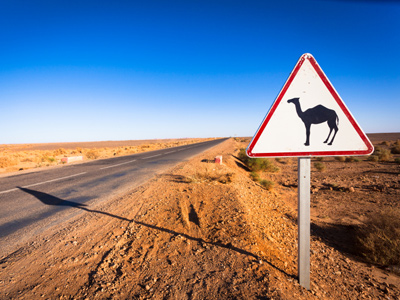This roadsign has the shape of a triangle.

# Level 3-4 Shapes - Shape Identification

Shapes - there are many you'll come across in KS3 Maths. Circles, triangles, squares, pentagons, rhomboids, trapeziums... the list goes on. But how good are you at shape identification?

Octagons and hexagons are different 2-Dimensional shapes but do you know what they look like? Some 2-Dimensional shapes are polygons whilst others are not, but do you know why? Some 2-Dimensional shapes are regular whilst others are irregular - can you tell the difference?

This quiz should be quite easy for you. It has a variety of two dimensional (2D) shapes that you will need to identify. See how many you recognise. It may seem very simple but please take your time and read each question thoroughly before you choose your answers. Over confidence can lead to carelessness and carelessness can lead to silly mistakes. And don't forget the helpful comments. If you do get any of the answers wrong then they should explain why.

1.
Can you pick out the hexagon in the picture below?
A
B
C
D
HeXagons have siX sides
2.
How many of the shapes below are polygons?
1
2
3
4
A polygon has at least 3 straight sides and angles
3.
From the shapes below, can you recognise the octagon?
A
B
C
D
It is in fact a regular octagon as all eight sides are of equal length and all internal angles are of the same size
4.
Which of the shapes below is a rectangle?
A
B
C
D
Rectangles have 4 straight sides and 4 right angles
5.
Which shape below is a circle?
A
B
C
D
Circles are round. Each point on their circumference is the same distance from their centre
6.
Which of the shapes below is NOT a polygon?
A
B
C
D
The circle does not have any straight sides or angles and is therefore not a polygon
7.
Which of the shapes below is the parallelogram?
A
B
C
D
Parallelograms are quadrilaterals with two pairs of parallel sides
8.
Which of the shapes below is a pentagon?
A
B
C
D
A pentagon is a 5-sided polygon
9.
Which of the shapes below is a triangle?
A
B
C
D
'Tri' means 3, as in 'tricycle'
10.
Which of the shapes below is the trapezium?
A
B
C
D
A trapezium is a quadrilateral with only one pair of parallel sides